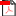Top Message Top Message
 Back to Home Page  |  Recommend a Site  |  Settings   |  Sign In Education Web 0 Chad 0 La. 0 Ohio 0 BCE 0 COPA Viewing 1-1 of 1 total resultsExercises for 3.7 Case Study: Population Modeling Exercises for 3.7 Case Study: Population Modeling algebraically, using the mathematical solution of the initial-value problem. With initial population 3.9 million in 1790 and 250 million in 1990, what value of k do you find using the algebraic methods? Give your answer accurate to 6 decimal places. What population do you project in the year 2350... 1 0 algebraically, using the mathematical solution of the initial-value problem. With initial population 3.9 million in 1790 and 250 million in 1990, what value of k do you find using the algebraic methods? Give your answer accurate to 6 decimal places. What population do you project in the year 2350 using the algebraic methods? million people How do the results of the algebraic methods compare with the values obtained experimentally using Eulers method? A. population growth is faster with Euler’s method B. population 1 0 http://www.math.dartmouth.edu/~klbooksite/3.07/307exercises.pdf#page=1 www.math.dartmouth.edu/~klbooksite/3.07/307exercises.pdf#page=1 algebraically, using the mathematical solution of the initial-value problem. With initial population 3.9 million in 1790 and 250 million in 1990, what value of k do you find using the algebraic methods? Give your answer accurate to 6 decimal places. What population do you project in the year 2350 using the algebraic methods? million people How do the results of the algebraic methods compare with the values obtained experimentally using Eulers method? A. population growth is faster with Euler’s method B. population www.math.dartmouth.edu/~klbooksite/3.07/307exercises.pdf#page=1 Average Rating (0 votes) © Copyright Vantage Labs LLC All rights reserved.    About iSEEK Education  |  Privacy Policy  |  Contact Us  |  FAQ Total time to process: 1646ms - Cache load: 0ms.